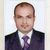# Connectivity & Data Prep

Discussion board where members can learn more about Qlik Sense Data Connectivity.

Announcements
Our May 2021 end-to-end product release from Data Integration to Data Analytics is out! READ DETAILS
cancel
Showing results for
Search instead for
Did you mean:Contributor III

## Max of Multiple fields in a table

Hi,

I am trying to get the maximum values for multiple fields is a table and display it in KPI.

for example : I have fields F1, F2, F3

F1 has values--> 1,3,4

F2 has values--> 1,2,3

F3 has values--> 2,3,4

so in this case KPI would show the maximum total of the 3 fields which is 9

can anyone please help.

Thank you.

1 Solution

Accepted SolutionsMVP

rangemax(sum(F1),sum(F2),sum(F3))

9 RepliesMVP

May be this

Sum(RangeMax(F1, F2, F3))MVP

How it should be 9 can you explain this please for understanding. Will it be maximum values for all three fields that is (3+3+3).Contributor III
Author

F1=1+2+4

F2=1+2+3

F3=2+3+4

the sum of F3 is the maximum this is what I want to showMVP

May be this

RangeMax(Sum(F1), Sum(F2), Sum(F3))MVP

rangemax(sum(F1),sum(F2),sum(F3))MVP

Where you want to use this expression.Contributor III
Author

Thank a lot this workedContributor III
Author

Thanks a lot this workedMVP

They are individual fields then try only this expression in any text object or the KPI object

=RangeMax(Sum(F1), Sum(F2), Sum(F3))

Or

=Sum(RangeMax(F1, F2, F3))